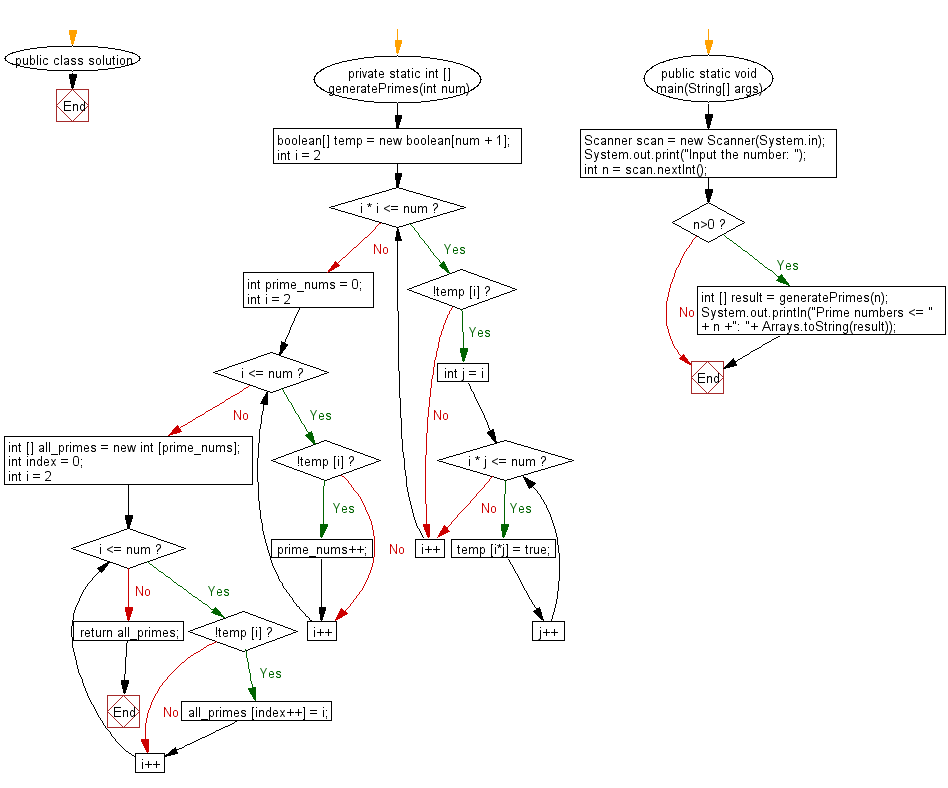﻿ Java Math Exercises: Print all primes smaller than or equal to any given number - w3resource# Java Math Exercises: Print all primes smaller than or equal to any given number

## Java Math Exercises: Exercise-20 with Solution

In mathematics, the sieve of Eratosthenes is an ancient algorithm for finding all prime numbers up to any given limit.
Write a Java program to print all primes smaller than or equal to any given number.

Sample Solution:

Java Code:

``````import java.util.*;

public class solution {

private static int [] generatePrimes(int num) {
boolean[] temp = new boolean[num + 1];
for (int i = 2; i * i <= num; i++) {
if (!temp [i]) {
for (int j = i; i * j <= num; j++) {
temp [i*j] = true;
}
}
}
int prime_nums = 0;
for (int i = 2; i <= num; i++) {
if (!temp [i]) prime_nums++;
}
int [] all_primes = new int [prime_nums];
int index = 0;
for (int i = 2; i <= num; i++) {
if (!temp [i]) all_primes [index++] = i;
}
return all_primes;
}

public static void main(String[] args)
{
Scanner scan = new Scanner(System.in);
System.out.print("Input the number: ");
int n = scan.nextInt();
if (n>0)
{
int [] result = generatePrimes(n);
System.out.println("Prime numbers <= " + n +": "+ Arrays.toString(result));
}
}
}
``````

Sample Output:

```Input the number:  125
Prime numbers <= 125: [2, 3, 5, 7, 11, 13, 17, 19, 23, 29, 31, 37, 41, 43, 47, 53, 59, 61, 67, 71, 73, 79, 83, 89, 97, 101, 103, 107, 109, 113]
```

Flowchart:Java Code Editor:

What is the difficulty level of this exercise?

﻿

## Java: Tips of the Day

Array vs ArrayLists:

The main difference between these two is that an Array is of fixed size so once you have created an Array you cannot change it but the ArrayList is not of fixed size. You can create instances of ArrayLists without specifying its size. So if you create such instances of an ArrayList without specifying its size Java will create an instance of an ArrayList of default size.

Once an ArrayList is full it re-sizes itself. In fact, an ArrayList is internally supported by an array. So when an ArrayList is resized it will slow down its performance a bit as the contents of the old Array must be copied to a new Array.

At the same time, it's compulsory to specify the size of an Array directly or indirectly while creating it. And also Arrays can store both primitives and objects while ArrayLists only can store objects.

Ref: https://bit.ly/3o8L2KH